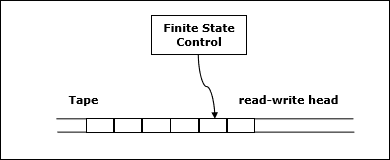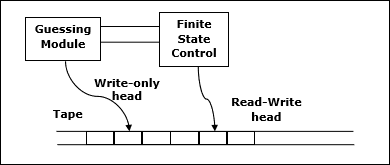• Design and Analysis of Algorithms
• Home

Deterministic vs. Nondeterministic Computations

To understand class P and NP, first we should know the computational model. Hence, in this chapter we will discuss two important computational models.

Deterministic Computation and the Class P

Deterministic Turing Machine

One of these models is deterministic one-tape Turing machine. This machine consists of a finite state control, a read-write head and a two-way tape with infinite sequence.

Following is the schematic diagram of a deterministic one-tape Turing machine.A program for a deterministic Turing machine specifies the following information −

• A finite set of tape symbols (input symbols and a blank symbol)
• A finite set of states
• A transition function

In algorithmic analysis, if a problem is solvable in polynomial time by a deterministic one tape Turing machine, the problem belongs to P class.

Nondeterministic Computation and the Class NP

Nondeterministic Turing Machine

To solve the computational problem, another model is the Non-deterministic Turing Machine (NDTM). The structure of NDTM is similar to DTM, however here we have one additional module known as the guessing module, which is associated with one write-only head.

Following is the schematic diagram.If the problem is solvable in polynomial time by a non-deterministic Turing machine, the problem belongs to NP class.

Useful Video Courses

Video

Compiler Design Online Training

102 Lectures 10 hours

Video

Sequential Circuit Design

30 Lectures 3 hours

Video

Design Patterns Online Training

31 Lectures 4 hours

Video

InDesign Online Training

43 Lectures 1.5 hours

Video

Canva: Become a Graphic Designer

7 Lectures 1 hours

Video

The Ultimate Canva Graphic Design Course

54 Lectures 4 hours